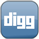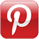### Simulating the Flip of a CoinIf you ever needed to simulate the flipping of a coin, or some other event in which you expect to receive a boolean result, this is how to do it.

When we last looked at generating random numbers, we looked at creating random numbers in a range. We'll use that code as a base. See below.
``` function coinFlip() {     var max = 1;     var min = 0;     var rndNum;     rndNum = min + Math.floor(Math.random() * ((max - min) + 1));     return ((rndNum == 0 ? true : false)); } ```
Of course, we can simplify the above code by removing the generic elements:
``` function coinFlip() {     return ((Math.floor(Math.random() * 2) == 0 ? true : false)); } ```
In the above code we generate our random number to get a 0 or 1, and then use a ternary operator to return true or false.